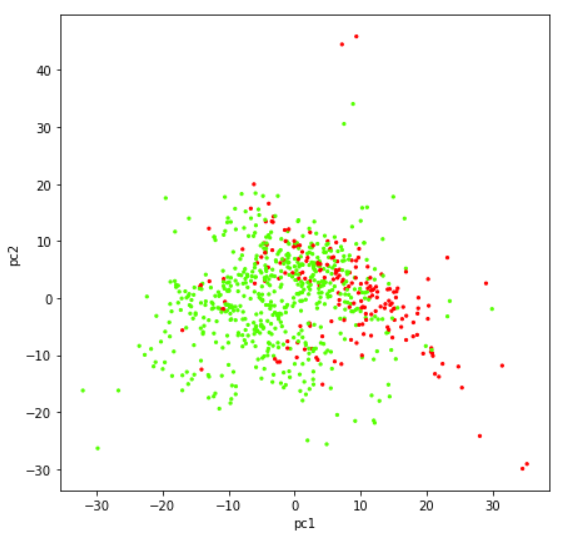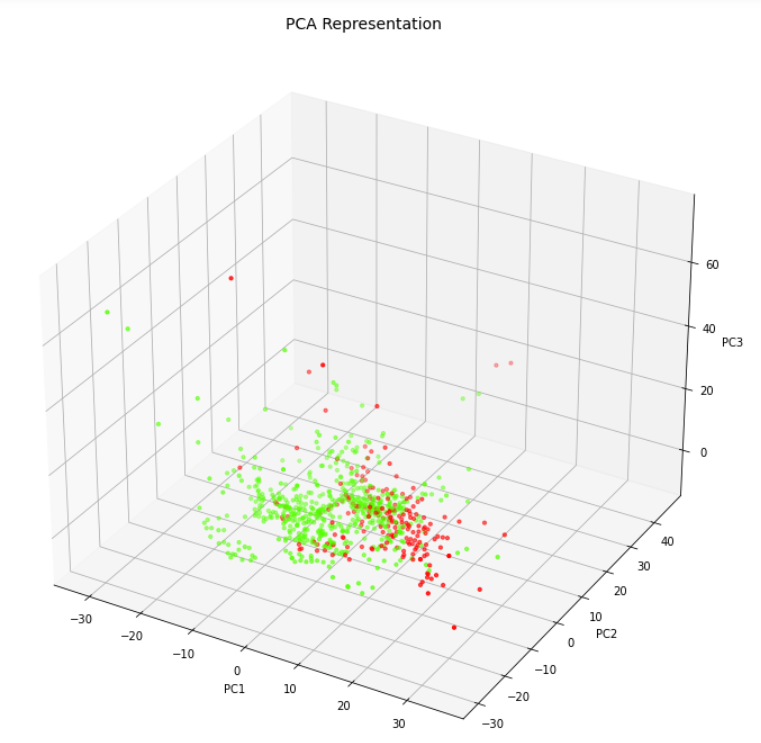# Complete Tutorial of PCA in Python Sklearn with ExampleWe are using a Parkinson’s disease dataset that contains 754 attributes and 756 records. As you can see it is highly dimensional with 754 attributes. It contains an attribute ‘class’ that contains 0 and 1 to denote the absence or presence of Parkinson’s disease.

### Importing necessary libraries

We first load the libraries required for this example.

In:

```from sklearn.decomposition import PCA
from sklearn.preprocessing import StandardScaler
from sklearn.model_selection import train_test_split
import pandas as pd
from sklearn.linear_model import LogisticRegression
from sklearn.metrics import accuracy_score
import matplotlib.pyplot as plt
import warnings
warnings.filterwarnings('ignore')```

Next, we read the dataset CSV file using Pandas and load it into a dataframe. We will do a quick check if the dataset got loaded properly by fetching the 5 records using the head function. We also validate the number of rows and columns by using shape property of the dataframe.

Finally, we calculate the count of the two classes 0 and 1 in the dataset.

In :

```df= pd.read_csv(r"pd_speech_features.csv")

gender PPE DFA RPDE numPulses numPeriodsPulses meanPeriodPulses stdDevPeriodPulses locPctJitter locAbsJitter tqwt_kurtosisValue_dec_28 tqwt_kurtosisValue_dec_29 tqwt_kurtosisValue_dec_30 tqwt_kurtosisValue_dec_31 tqwt_kurtosisValue_dec_32 tqwt_kurtosisValue_dec_33 tqwt_kurtosisValue_dec_34 tqwt_kurtosisValue_dec_35 tqwt_kurtosisValue_dec_36 class
0 1 0.85247 0.71826 0.57227 240 239 0.008064 0.000087 0.00218 0.000018 1.5620 2.6445 3.8686 4.2105 5.1221 4.4625 2.6202 3.0004 18.9405 1
1 1 0.76686 0.69481 0.53966 234 233 0.008258 0.000073 0.00195 0.000016 1.5589 3.6107 23.5155 14.1962 11.0261 9.5082 6.5245 6.3431 45.1780 1
2 1 0.85083 0.67604 0.58982 232 231 0.008340 0.000060 0.00176 0.000015 1.5643 2.3308 9.4959 10.7458 11.0177 4.8066 2.9199 3.1495 4.7666 1
3 0 0.41121 0.79672 0.59257 178 177 0.010858 0.000183 0.00419 0.000046 3.7805 3.5664 5.2558 14.0403 4.2235 4.6857 4.8460 6.2650 4.0603 1
4 0 0.32790 0.79782 0.53028 236 235 0.008162 0.002669 0.00535 0.000044 6.1727 5.8416 6.0805 5.7621 7.7817 11.6891 8.2103 5.0559 6.1164 1

5 rows × 754 columns

In :

`df.shape`

Out:

`(756, 754)`

### Applying PCA with Principal Components = 2

Now let us apply PCA to the entire dataset and reduce it into two components. We are using the PCA function of sklearn.decomposition module.

After applying PCA we concatenate the results back with the class column for better understanding.

In :

```pca2 = PCA(n_components=2)
principalComponents = pca2.fit_transform(X_Scale)

principalDf = pd.DataFrame(data = principalComponents, columns = ['principal component 1', 'principal component 2'])

finalDf = pd.concat([principalDf, df[['class']]], axis = 1)

Out:

principal component 1 principal component 2 class
0 -10.184156 1.252252 1
1 -10.621219 1.659891 1
2 -13.507782 -1.231873 1
3 -9.277452 8.087223 1
4 -7.142122 3.815401 1

### Visualizing Data in 2 Dimension Scatter Plot

Let us now visualize the dataset that has been reduced to two components with the help of a scatter plot.

In :

```plt.figure(figsize=(7,7))
plt.scatter(finalDf['principal component 1'],finalDf['principal component 2'],c=finalDf['class'],cmap='prism', s =5)
plt.xlabel('pc1')
plt.y;label('pc2')```### Applying PCA with Principal Components = 3

Just like earlier, let us again apply PCA to the entire dataset to produce 3 components.

In :

```pca3 = PCA(n_components=3)
principalComponents = pca3.fit_transform(X_Scale)

principalDf = pd.DataFrame(data = principalComponents, columns = ['principal component 1', 'principal component 2', 'principal component 3'])

finalDf = pd.concat([principalDf, df[['class']]], axis = 1)

Out:

principal component 1 principal component 2 principal component 3 class
0 -10.184156 1.252253 -7.185881 1
1 -10.621219 1.659890 -6.873706 1
2 -13.507782 -1.231873 -7.076563 1
3 -9.277453 8.087221 14.467958 1
4 -7.142122 3.815398 15.474813 1

### Visualizing Data in 3 Dimension Scatter Plot

Let us visualize the three PCA components with the help of 3-D Scatter plot.

In :

```from mpl_toolkits.mplot3d import Axes3D

fig = plt.figure(figsize=(9,9))
axes = Axes3D(fig)
axes.set_title('PCA Representation', size=14)
axes.set_xlabel('PC1')
axes.set_ylabel('PC2')
axes.set_zlabel('PC3')

axes.scatter(finalDf['principal component 1'],finalDf['principal component 2'],finalDf['principal component 3'],c=finalDf['class'], cmap = 'prism', s=10)```

Out:## 6. Improve Speed and Avoid Overfitting of ML Models with PCA using Sklearn

Now we will see the curse of dimensionality in action. We will create two logistic regression models – first without applying the PCA and then by applying PCA. We will capture their training times and accuracies and compare them.

Here we are going to separate the dependent label column into y dataframe. And all remaining columns into X dataframe.

Then we split them into train and test sets in ration of 70%-30% using train_test_split function of Sklearn.

In :

```X = df.drop('class',axis=1).values
y = df['class'].values

X_train, X_test, y_train, y_test = train_test_split(X,y, test_size=0.3,random_state=0)```

### Standardizing the Dataset

This time we apply standardization to both train and test datasets but separately.

In :

```scaler = StandardScaler()

# Fit on training set only.
scaler.fit(X_train)

# Apply transform to both the training set and the test set.
X_train_pca = scaler.transform(X_train)
X_test_pca = scaler.transform(X_test)```

### Creating Logistic Regression Model without PCA

Here we create a logistic regression model and can see that the model has terribly overfitted. The training accuracy is 100% and the testing accuracy is 84.5%.

Also do keep a note that the training time was 151.7 ms here.

In :

```%%time

logisticRegr = LogisticRegression()
logisticRegr.fit(X_train,y_train)

y_train_hat =logisticRegr.predict(X_train)
train_accuracy = accuracy_score(y_train, y_train_hat)*100
print('"Accuracy for our Training dataset with PCA is: %.4f %%' % train_accuracy)

```

Out:

```"Accuracy for our Training dataset with PCA is: 100.0000 %
Wall time: 151.7 ms```

In :

```y_test_hat=logisticRegr.predict(X_test)
test_accuracy=accuracy_score(y_test,y_test_hat)*100
test_accuracy
print("Accuracy for our Testing dataset with tuning is : {:.3f}%".format(test_accuracy) )```

Out:

`Accuracy for our Testing dataset with tuning is : 84.581%`

### Creating Logistic Regression Model with PCA

Below we have created the logistic regression model after applying PCA to the dataset. It can be seen that this time there is no overfitting with the PCA dataset. Both training and the testing accuracy is 79% which is quite a good generalization.

```%%time

logisticRegr = LogisticRegression()
logisticRegr.fit(X_train_pca,y_train)

y_train_hat =logisticRegr.predict(X_train_pca)
train_accuracy = accuracy_score(y_train, y_train_hat)*100
print('"Accuracy for our Training dataset with PCA is: %.4f %%' % train_accuracy)```